# How do I insert math equations into my lesson plans?

This is a feature available to Chalk Gold subscribers and teachers whose schools are subscribed to our paid Chalk solutions. You will not have this option if you are a free user.

Including math equations in your lessons can be valuable for comprehension and delivery for STEM-based subjects, but can be difficult to include through plain text. This article will show you how to insert math equations and formulas directly into your lessons through our lesson editor.

Note: This support article goes over how to use the math editor in the context of your lesson planning, but if your school is subscribed to our Curriculum option then you will be able to use the editor within your curriculum maps as well.

## Step 1:

Open a lesson you would like to insert math equations for and click the math editor icon in the formatting menu, as shown below.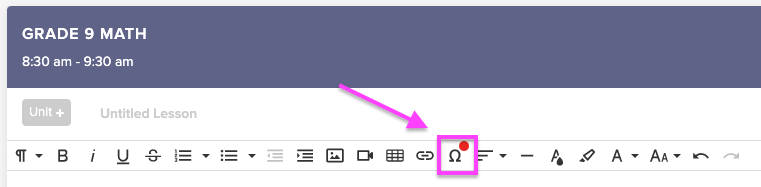## Step 2:

When you first open the math editor, it will default to the Graphical Editor option. Here you can use your keyboard inputs to enter simple codes and functions and see a visual demonstration of how they will appear when you insert them into your lessons.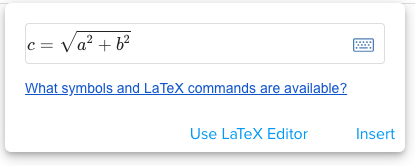Click the icon to the right of the input field to toggle a virtual keyboard that you can use to input various commands.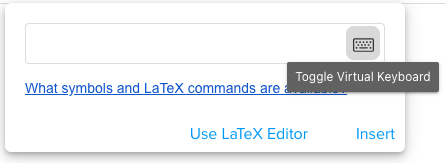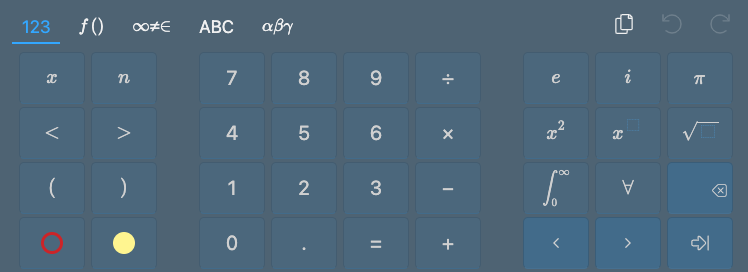You can click the Use LaTeX Editor button to switch to a LaTeX command input format. For more information on using LaTeX commands in the math editor, please read the "What LaTeX commands are available?" section at the bottom of this support article.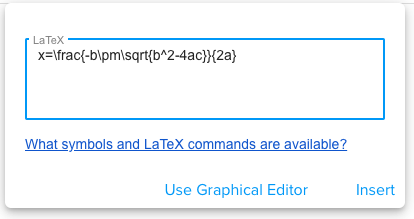Note: You can also use the editor for chemical equations by using the \ce LaTeX command, such as "\ce{H2O}" shown below.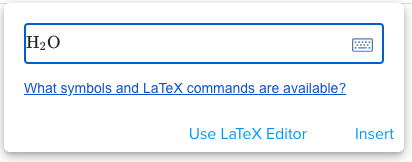## Step 3:

When you've completed entering the content, click Insert and it will be embedded into your lesson as an image.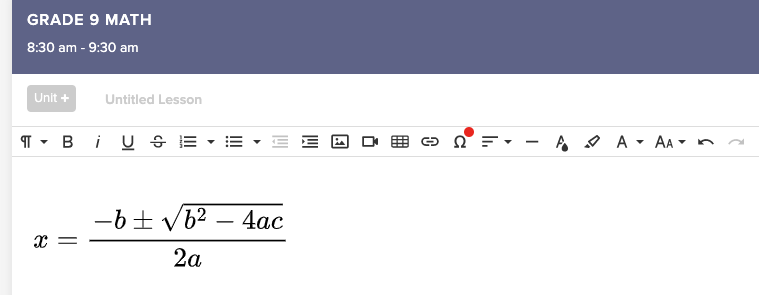## Step 4:

Once you've inserted a math equation into your lesson, you can click on it to display a menu of available editing options. You can resize the image, change display options, add captions, or edit the equation contents as necessary.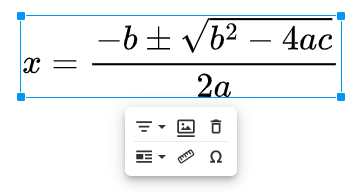Unfortunately, this feature is not yet available for our mobile apps.

Unfortunately, this feature is not yet available for our mobile apps.

# What LaTeX commands are available?

LaTeX is a command system for technical or mathematical typesetting that is used by mathematicians worldwide. You can use the LaTeX option in the math editor to embed complex mathematical formulas directly into your lesson plans.

The math editor feature supports over 800 LaTeX commands. For a full list of supported LaTeX commands, please see the following article.

Here are some commonly-used commands:

Operators

• Times (×): \times
• Dot (⋅): \cdot
• Division (÷): \div
• Plus minus (±): \pm

Commands

• Fractions: \frac{1}{2}
• Nested fractions: \frac{\frac{1}{2}}{2}
• Square root (√3): \sqrt[root]{}

Relations

• Not equal (≠): \neq
• Approximately equal (≈): \approx
• Less than or equal (≤): \leq
• Greater than or equal (≥): \geq
• Much less than (≪): \ll
• Much greater than (≫): \gg

Exponents/Indices

• Use ^ for superscript. Example: x^2
• Use ^{} for exponents with >1 digit. Example: x^{10}
• Use _ for subscript. Example: x_0
• Use _{} for subscript with >1 digit. Example: x_{10}

Other Symbols

• Infinity (∞): \infty
• Partial (∂): \partial
• Estimator (θ̂): \hat{}

Logic

• For all (∀): \forall
• Exists (∃): \exists
• Or (∨): \lor
• And (∧): \land
• Xor (⊻): \veebar
• Not (¬): \neg

Sets (You can put an "n" before the command to get the negation)

• Proper superset (⊃): \supset
• Superset (⊇): \supseteq
• Proper Subset (⊂): \subset
• Subset (⊆): \subseteq
• Member (∈): \in
• Empty set (∅): \emptyset
• Set of real numbers (ℝ): \mathbb{R}
• Set union (belonging to A OR B) (∪): \cup
• Set intersection (belonging to A AND B) (∩): \cap

Greek Letters (You can capitalize the symbol by capitalizing the command)

• alpha (α): \alpha
• beta (β): \beta
• gamma (γ): \gamma
• delta (δ): \delta
• epsilon (ϵ): \epsilon
• zeta (ζ): \zeta
• eta (η): \eta
• theta (θ): \theta
• iota (ι): \iota
• kappa (κ): \kappa
• lambda (λ): \lambda
• mu (μ): \mu
• nu (ν): \nu
• xi (ξ): \xi
• omicron (o): o
• pi (π): \pi
• rho (ρ): \rho
• sigma (σ): \sigma
• tau (τ): \tau
• upsilon (υ): \upsilon
• phi (ϕ): \phi
• chi (χ): \chi
• psi (ψ): \psi
• omega (ω): \omega